## alpha_stable

Sample and generate probability distribution functions of Alpha-Stable distributions in Rust

### 2 releases

 0.1.1 Nov 18, 2023 Nov 4, 2023

#591 in Math

620KB
364 lines

# alpha-stable

Sample and generate probability distribution functions of Alpha-Stable distributions in Rust.

## Specifying the distribution

Distributions are specified using one of two forms:

• Standard form: S_alpha(sigma, beta, mu) - equivalent to the 'first parameterization' of Wikipedia with c = sigma.
• Nolan's form: S^0_alpha(sigma, beta, mu_0) - equivalent to the 'second parameterization' in Wikipedia with delta = mu_0 and gamma = sigma.

## Examples: random walks

Here are three random walks generated by examples/distribution/main.rs

### Alpha = 2 - a normal distribution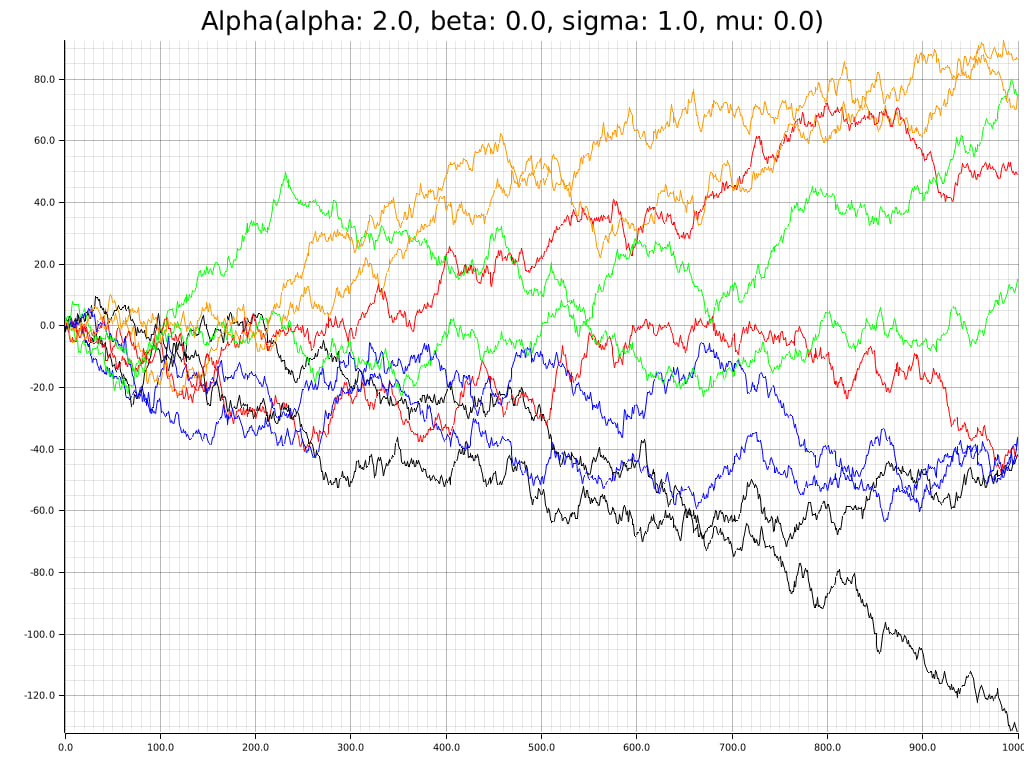### Alpha = 1.5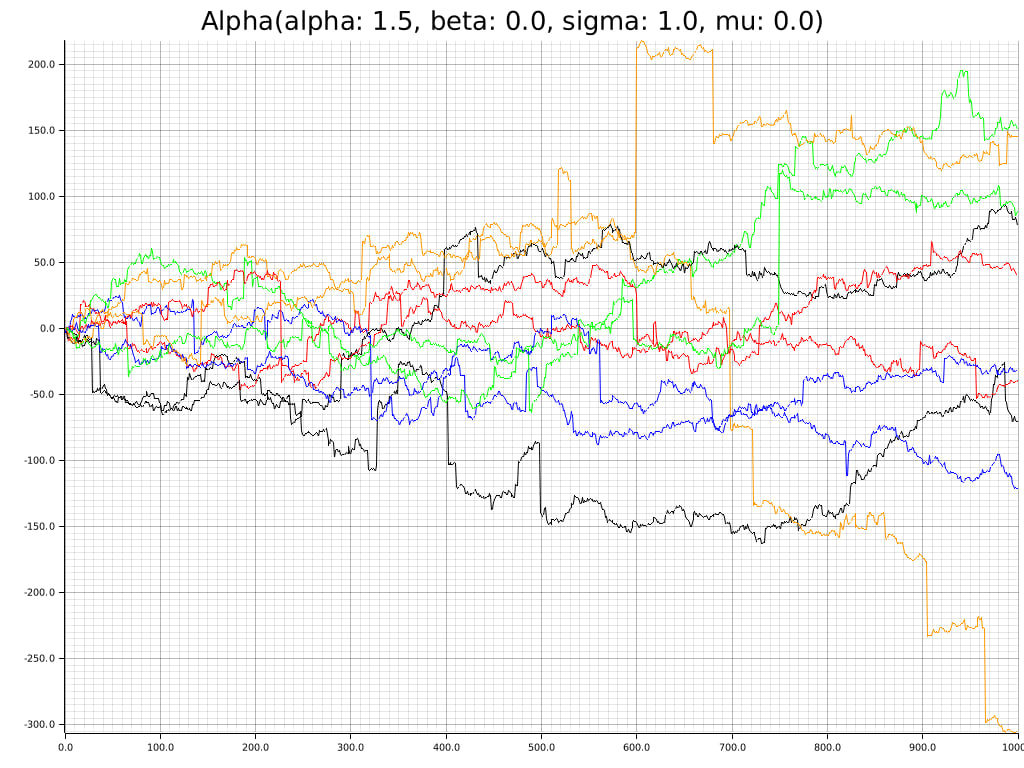### Alpha = 1.1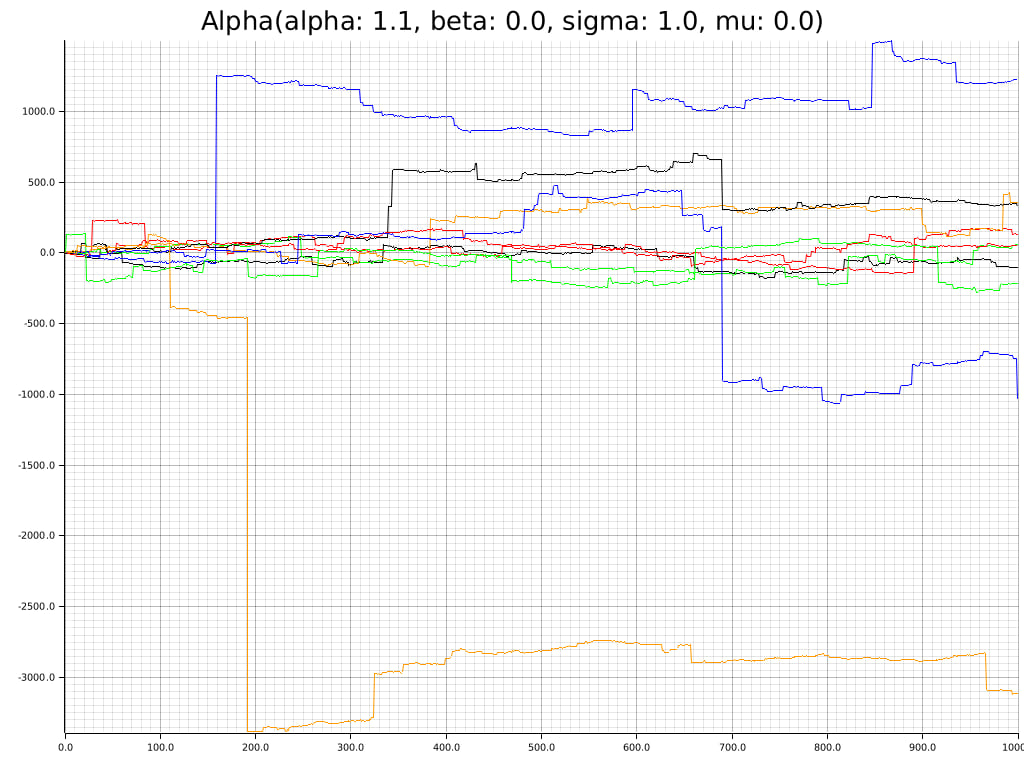## Examples: distributions

A set of probability distribution functions specified in Standard and Nolan's forms.

### Standard form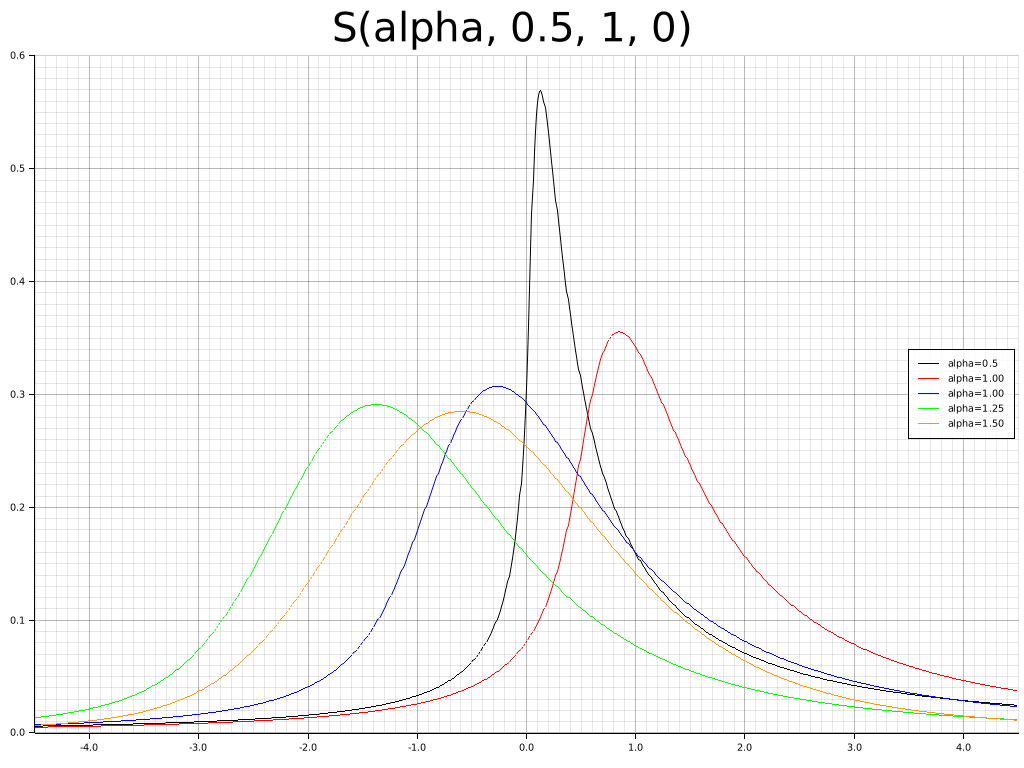### Nolan's form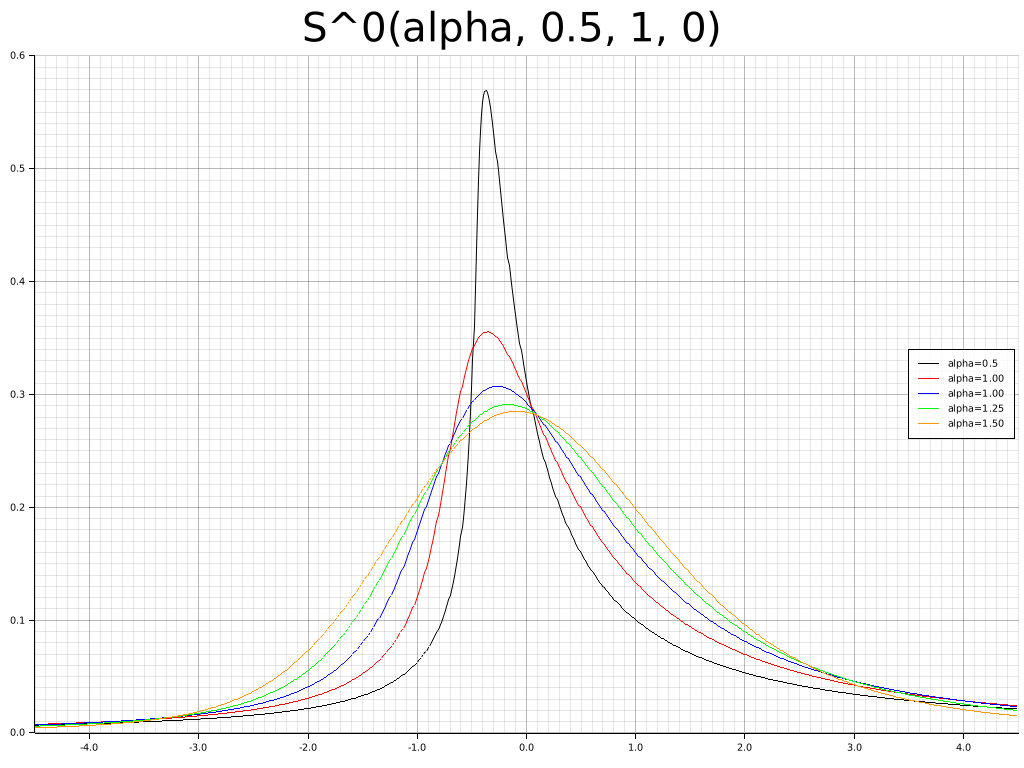## Examples: sample histograms

Some sample histograms to show samples and probability distribtions are consistent.

### Alpha = 1.1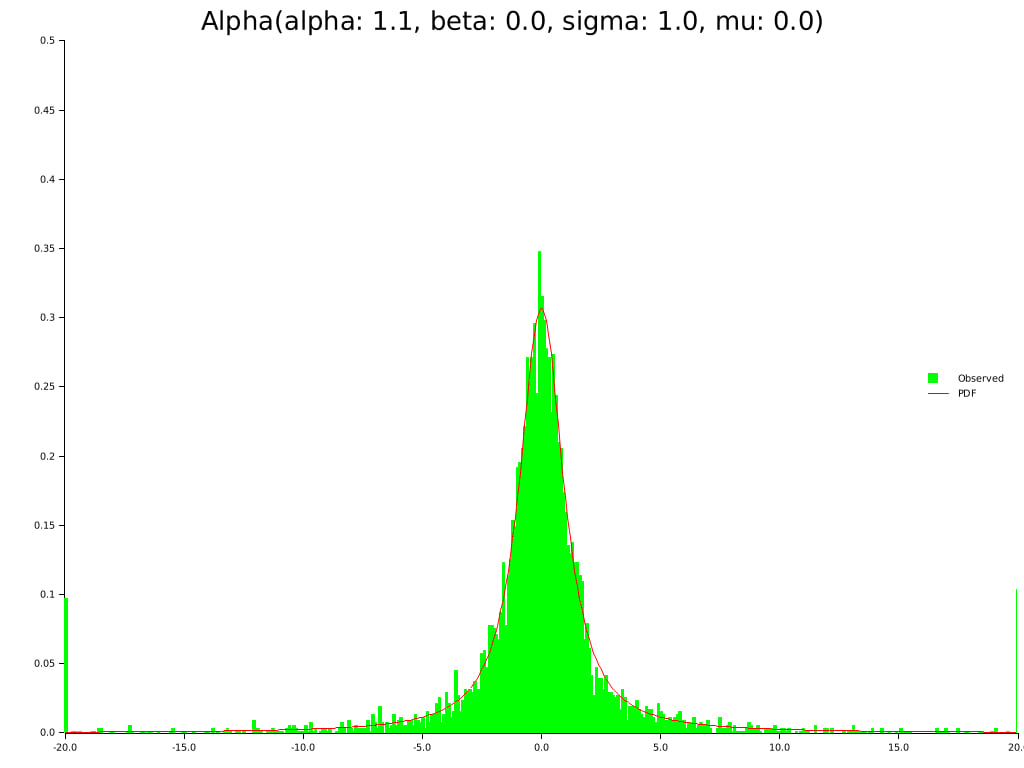### Alpha = 1.5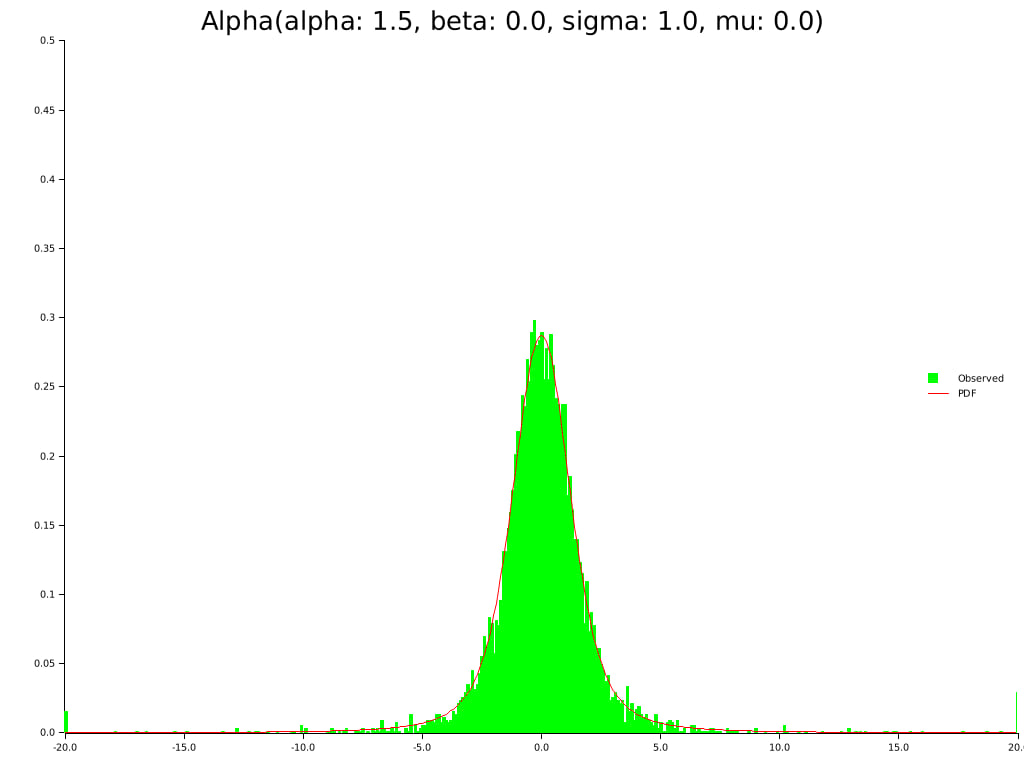### Alpha = 2 - a normal distribution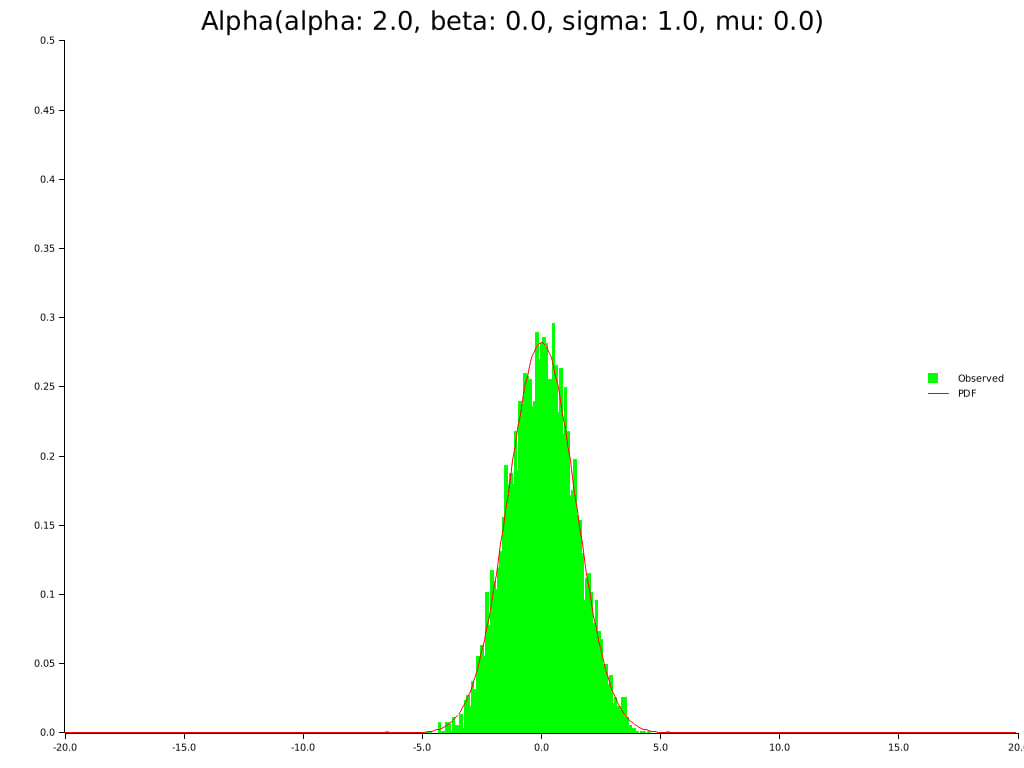### Alpha = 1.1; Beta = 0.5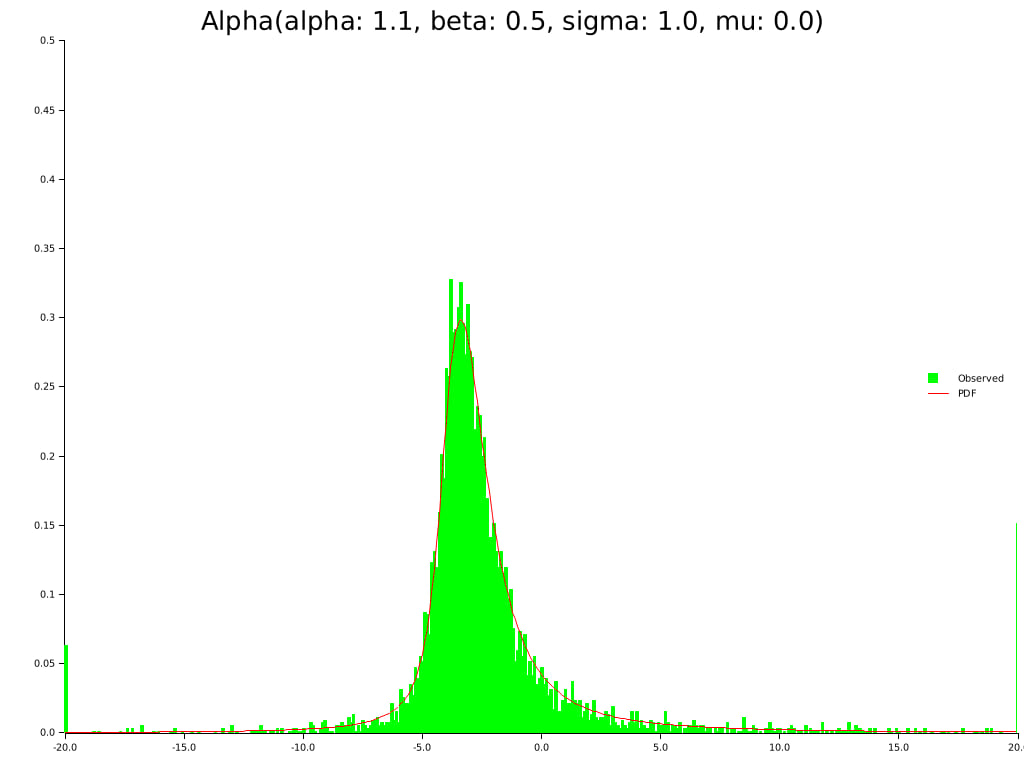~1.7–2.4MB
~53K SLoC